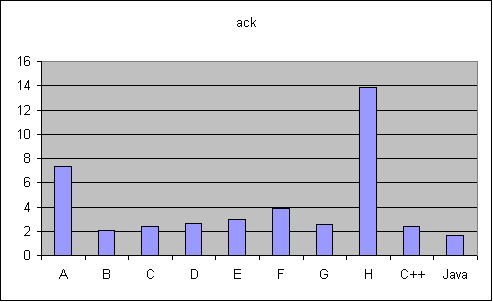# Ackermann's Function

Computes Ackermann's function, A(x, y), for x = 3 and y = N.  This function is a simple example of a total function that is computable but not primitive recursive. The function f(x) = A(x, x) grows much faster than polynomials or exponentials.  A(x, y) is defined as:

```        1. If x = 0 then  A(x, y) = y + 1
2. If y = 0 then  A(x, y) = A(x-1, 1)
3. Otherwise,     A(x, y) = A(x-1, A(x, y-1))```

Computing A(3, k) = 2(k+3) - 3 requires at least 4(k+1) function calls, and reaches a recursive depth of 2(k+3) -1.  Thus computation of it stresses your compiler's ability to do deep recursion efficiently.  In particular, it tests your code generation for function calls.  If you happened to implement tail-call elimination you will see a very large performance gain.

Source: [ack.im ack.cpp Ack.java]
Input: N=10
Output:
A(3,10) = 8189

 Java 1.63B 2.03 C++ 2.36 C 2.38 G 2.54 D 2.62 E 2.99 F 3.89 A 7.34 H 13.88